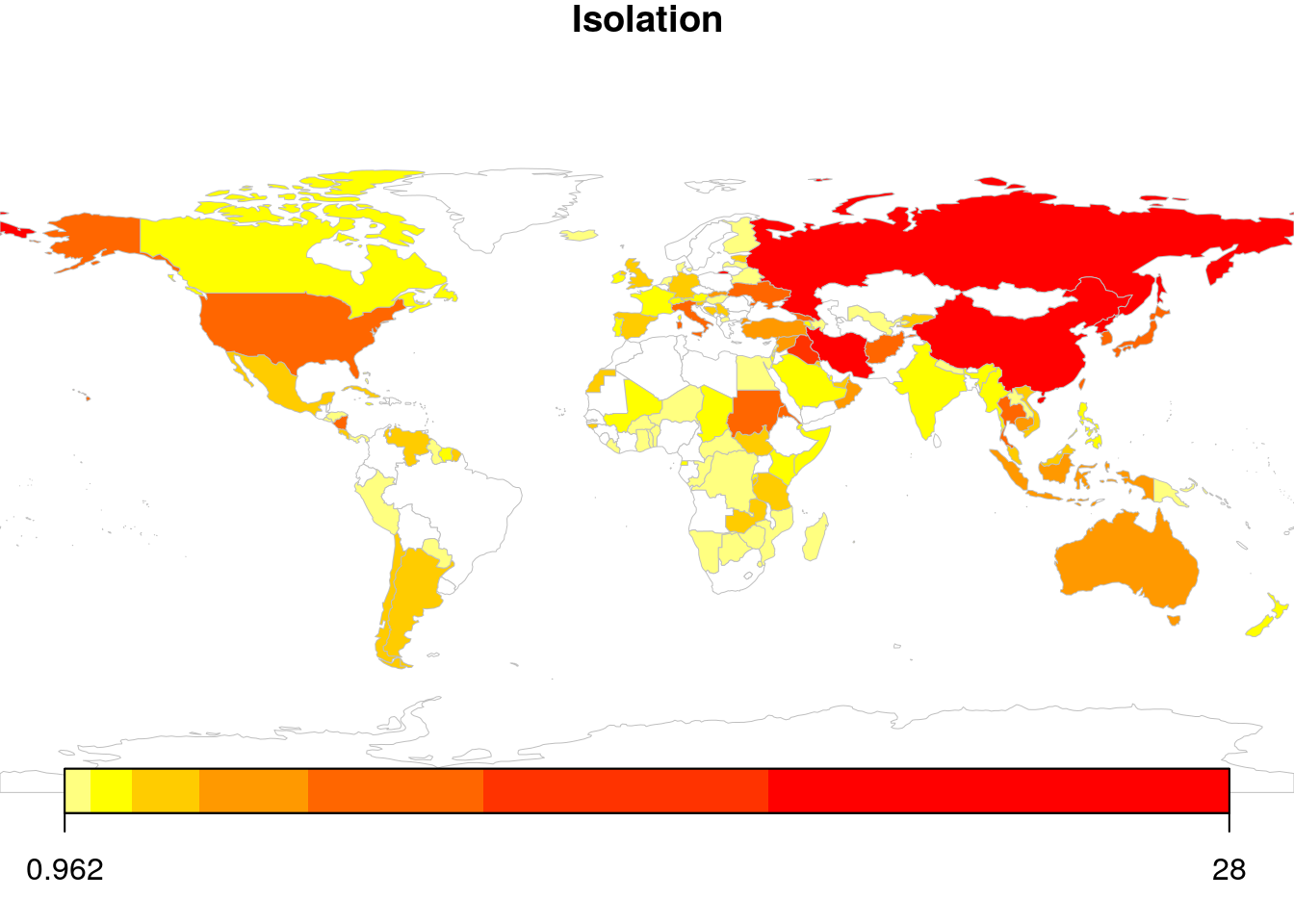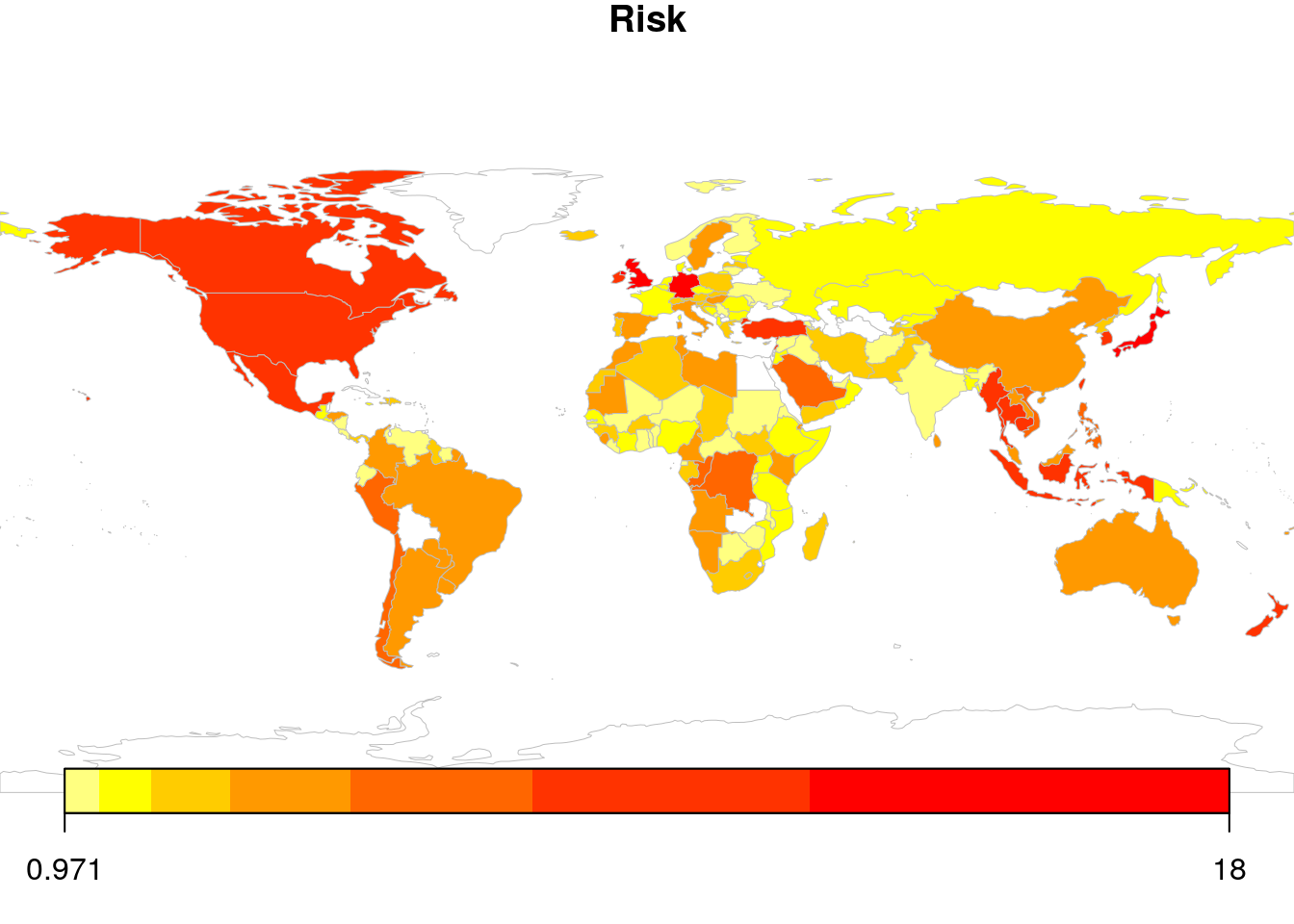# Scraping and visualising global news heatmaps with RIt’s nothing fancy, but here’s the code.It’s nothing fancy, but here’s the code.

library(rvest) # Web scraping
library(rworldmap) # Heatmap

Then I load a table of country names and ISO 3 codes (copied from Wikipedia, with some edits):

countries <- read.csv("countries.csv")
countries <- data.frame(Name = countries[,2], ISO3 = countries[,1])

Now I create a few keywords which relate to my chosen topics. This is a very rudimentary approach, but given the fact that I cannot download the article contents, it seems that a simpler method is more appropriate:

terms <- list(
Growth = c("boom", "expand", "growing", "to grow", "accelerat"),
Decline = c("bust", "depression", "recession", "downturn", "slowdown", "decline"),
Inflation = c("devalu", "inflat", "price"),
Isolation = c("tariff", "trade war", "sanction"),
Risk = c("risk", "uncertain", "volatil", "unknown")
)

terms_mat <- matrix(NA, nrow(countries), length(terms))

Google News provides headlines and summary texts from different news sources matching a given search query. Using rvest I extract the top 100 headlines and summary texts for a each country, where the search query is simply country-name economy (e.g. US economy). rvest makes scraping the news articles extremely simple. Subsequently, I count the number of articles mentioning each of the keywords relating to the topics:

for (i in 1:nrow(countries)) {

name <- countries[i, "Name"] %>% tolower %>% gsub(" ", "%20", .)
title <- content %>% html_nodes('div.mEaVNd') %>% html_text() %>% tolower
scrape.results[[as.character(countries[i,"ISO3"])]] <- title

for (j in 1:length(terms)) {

temp.sum <- 0
for (k in 1:length(terms[[j]])) {
temp.sum <- temp.sum + sum(sapply(scrape.results[[i]], FUN = function(x) grepl(terms[[j]][k], x)))
}
terms_mat[i,j] <- temp.sum / length(scrape.results[[i]]) * 100

}

}

Now all that remains is to plot the results. Here’s the plot for the topic of risk / uncertainty:

topic <- "Risk"

map.df <- cbind(countries, terms_mat)
colnames(map.df) <- c(colnames(countries), names(terms))
map.df[map.df==0] <- NA

map.data <- joinCountryData2Map(map.df, joinCode = "ISO3", nameJoinColumn = "ISO3")

save(map.data, file = "map_data.RData")

par(mai=c(0,0,0.2,0),xaxs="i",yaxs="i")
mapCountryData(map.data, nameColumnToPlot = topic, catMethod = "logFixedWidth", colourPalette = "heat",
missingCountryCol = "white")The results are extremely interesting and range from a few no-brainers (UK, Turkey, US) to some real surprises (New Zealand, Germany, Indonesia). Of course, these results might not surprise someone more versed in the current affairs of these countries, but for the rest of us, this map can help to create a more comprehensive understanding of the current global economic landscape.

Here’s the map for economic busts: